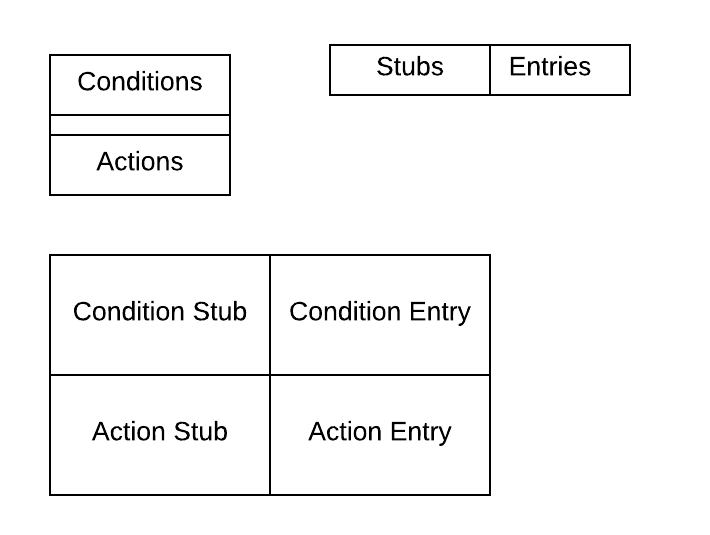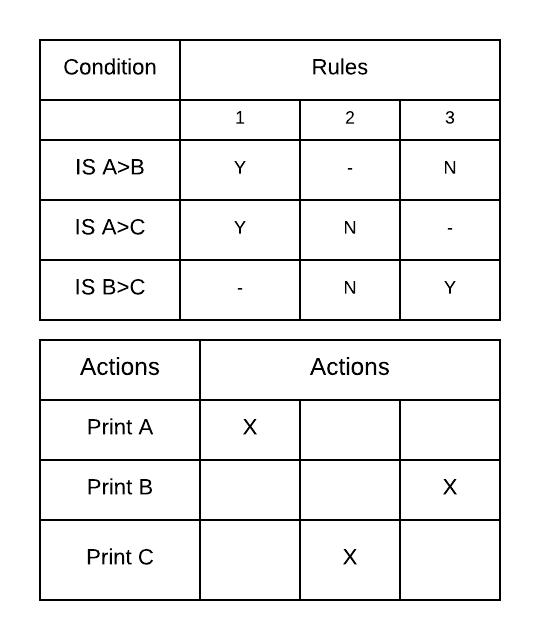October 3, 2023Decision Tables: Decision tables are powerful tools for expressing complex logical relationships in an understandable manner. They are used to define clearly & concisely the decision statement of a problem in a tabular form. A decision table is a table of conditions for defining a problem & the actions to be taken.

The table is divided into two horizontal & two vertical parts resulting in four distinct areas quadrants

The quadrants are usually separated by double lines

The left hand of the upper portion called condition stub deals with the given conditions

The right hand of upper portion called condition entry deals with the truth value of a condition. If the condition is true then mark Y against it otherwise N.

The left-hand side of the lower portion of the decision table is called action stub & this portion enlists all the possible actions.

The right-hand side of the lower portion is called Action Entry. If an action is to be taken depending upon the upper portion of the table then a mark (Y) or a (X) is written in the appropriate column otherwise it is left blank.## Steps in Writing Decision Tables

1. Define the problem accurately that has to be solved by a computer.
2. List out all the conditions to be tested in the problem.
3. List out the corresponding actions that should be taken with each combination of conditions.
4. Form a decision table using the two lists.

## Types of Decision Tables

1. ### Limited Entry Tables

If the stub portion completely defines the condition (or action), it is called a limited entry table.

1. ### Extended Entry Tables

If the stub portion does not completely express the condition (or action) then it is called an extended entry table.

Eg.

Develop a decision table to print the greatest of the three unequal positive numbers.3

There are 3 conditions & thus 2    = 8 possible rules. Out of 8 rules, two rules are logically impossible. The other rules take care of all possibilities. A don’t care entry (-) corresponds to both true & false condition.

Eg.

A rule [Y,Y] will split up into two rules [YYN] and [YYY]

1. When the condition are numerous, then the decision table help to visualize the outcomes of a situation
2. Decision tables summarize all the outcomes of a situation & suggest suitable actions
3. They provide more compact documentation
4. Decision tables can be changes easily.
5. Decision table have a standard format.

## Rules Should be followed in Constructing Decision Table

1. A decision should be given a name, shown in the top left of the table.
2. The logic of the decision table is independent on the sequence in which the condition rules are written, but the action takes place in the order in which the events occur.
3. Standardized language must be used consistently.
4. Duplication of terms & meaning should be eliminated where possible.

## Limitations

1. Decision tables only present a partial solution
2. Do not depict the flow of logic of a solution
3. Decision tables are quite far away from high level languages

Hope you liked this Article. Keep Visiting Mentor Way for more Articles like this.